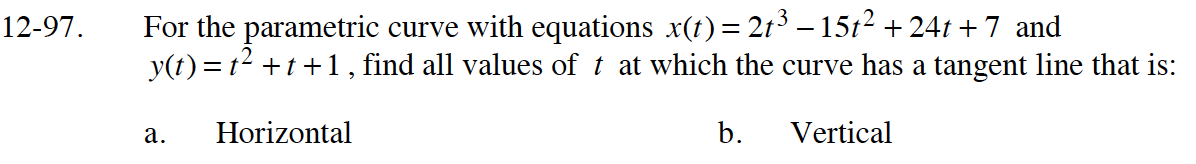### Home > CALC > Chapter 12 > Lesson 12.2.3 > Problem12-97

12-97.
1. For the parametric curve with equations x(t) = 2t3 − 15t2 + 24t + 7 and y(t) = t2 +t +1, find all values of t at which the curve has a tangent line that is: Homework Help ✎

1. Horizontal

2. VerticalIf the tangent line is horizontal, the dy = 0.
If the tangent line is vertical, then dx = 0.